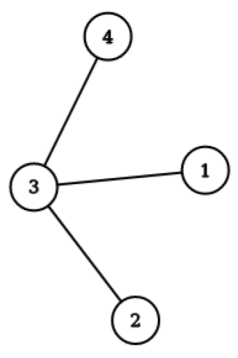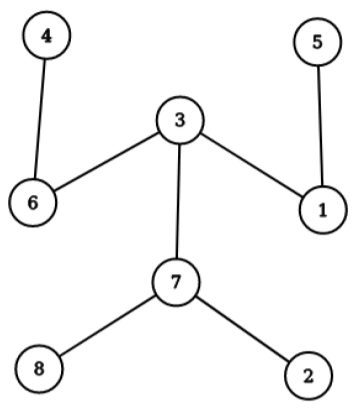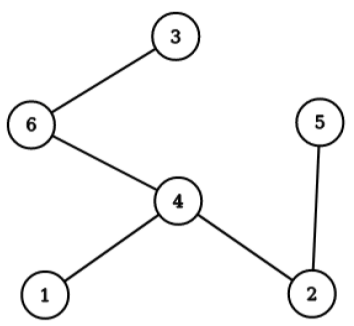시간 제한메모리 제한제출정답맞은 사람정답 비율
10 초 1024 MB0000.000%

## 문제

On the beautiful mountain of Velebit there are $N$ shelters. Exactly $N - 1$ pairs of shelters are connected by a hiking path such that it is possible to travel between any pair of shelters using these paths.

Vila the Fairy likes hiking very much and it takes her exactly one day to traverse a hiking path connecting two shelters. She can use her magical abilities to appear at any shelter at the beginning of a day, and then spend the next $K - 1$ days hiking in such a way that she never visits the same shelter more than once. Thus, during her hike, Vila visits exactly $K$ shelters.

Vila gets thirsty while hiking so she would like some shelters to have water wells. During any possible hike of hers she wants to visit exactly one shelter with a well.

Your task is to determine whether it is possible to find a subset of shelters at which to put wells to satisfy Vila’s peculiar wishes. In addition, you need to calculate the number of such subsets modulo 109 + 7.

Formally, given a tree of $N$ vertices and a positive integer $K$, determine if there is a subset of vertices such that any path containing exactly $K$ vertices has exactly one vertex from the subset. Additionally, you are asked to find the number of such subsets modulo 109 + 7.

## 입력

The first line contains two integers $N$ and $K$ ($2 \le K \le N$) from the task description.

The next $N - 1$ lines describe the hiking paths. The $i$-th of these lines contains two space-separated integers $a_i$ and $b_i$ ($1 \le a_i, b_i \le N$), representing a hiking path between shelters $a_i$ and $b_i$.

It is guaranteed that these paths form a tree.

## 출력

On the first line, output "YES" if there exists a subset of shelters satisfying Vila’s conditions and "NO", otherwise.

On the second line output the number of possible subsets of shelters satisfying Vila’s conditions modulo 109 + 7.

## 서브태스크

번호 배점 제한
1 30

$2 \le K \le N \le 200$

2 20

$2 \le K \le N \le 10\,000$

3 20

$2 \le K \le N \le 500\,000$

4 30

$2 \le K \le N \le 1\,500\,000$

If your program outputs the correct first line, but does not provide the correct second line, that test case will be scored with 60% of points allocated for the subtask it is part of.

The score for each subtask equals the smallest score obtained by one of its test cases.

## 예제 입력 1

4 2
3 4
3 1
2 3


## 예제 출력 1

YES
2The valid subsets of shelters are {3} and {1, 2, 4}.

## 예제 입력 2

8 3
7 3
1 3
7 8
5 1
4 6
7 2
3 6


## 예제 출력 2

NO
0## 예제 입력 3

6 5
4 1
4 2
3 6
5 2
4 6


## 예제 출력 3

YES
10There is only one path of length 5, and that path contains nodes 3, 6, 4, 2, and 5. Exactly one of these nodes must be in a sought subset, and it makes no difference whether node 1 is in the subset.

Therefore, the valid subsets of shelters are {3}, {1, 3}, {6}, {1, 6}, {4}, {1, 4}, {2}, {1, 2}, {5}, {1, 5}.

## 채점 및 기타 정보

• 예제는 채점하지 않는다.